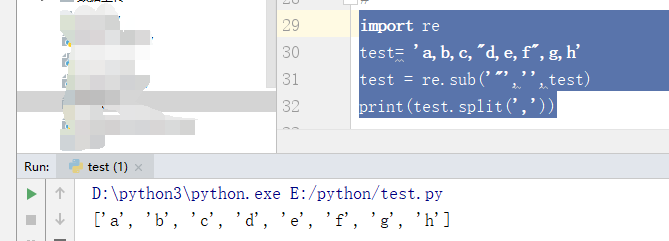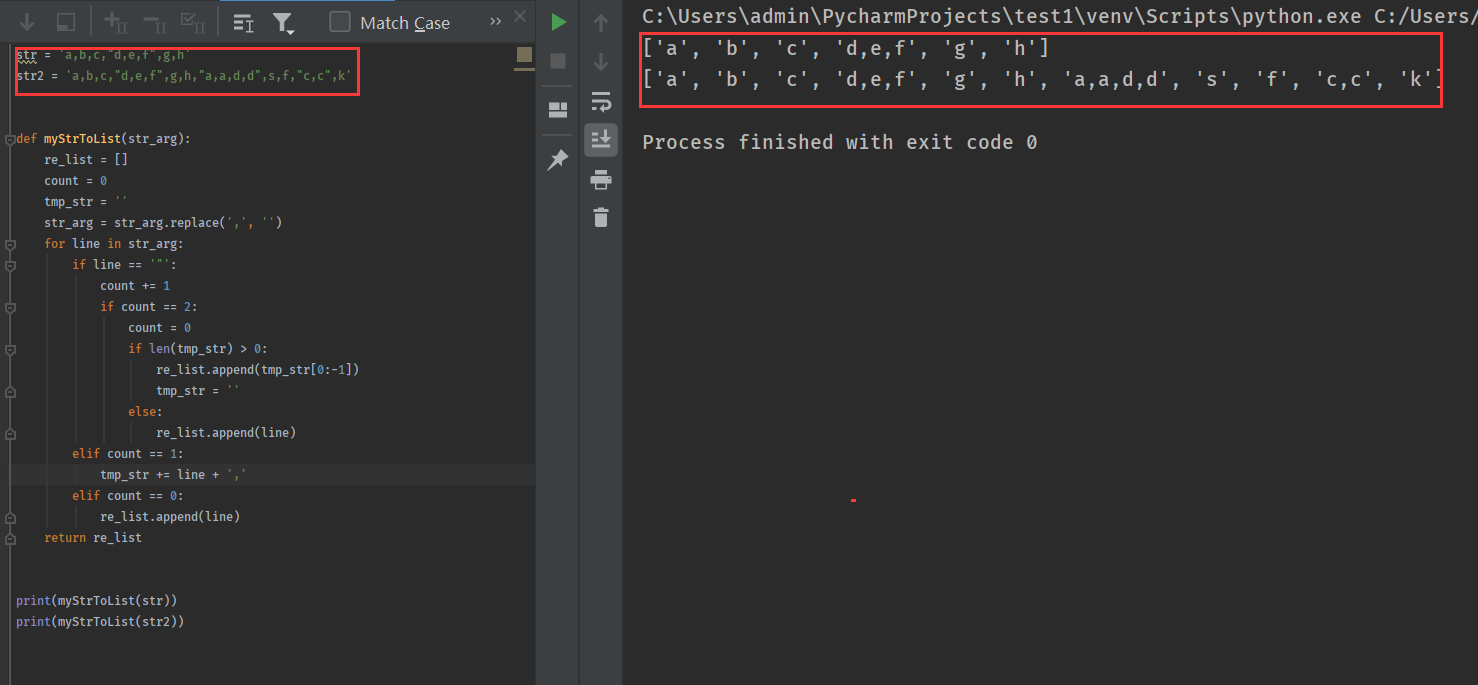Python关于将字符串进行分割并返回成一个列表？？？

4个回答SUN小圣 我是刚学的，还不熟练，可以具体一点吗？
5 个月之前 回复

``````import re
test= 'a,b,c,"d,e,f",g,h'
test = re.sub('"','',test)
print(test.split(','))
``````SUN小圣 可能我说的有问题，我想得到的列表是['a','b','c','d,e,f','g','h']，而不是['a',b','c','d','e','f','g','h']
5 个月之前 回复
``````import csv
str='a,b,c,"d,e,f",g,h'
newStr = ['{}'.format(x) for x in list(csv.reader([str], delimiter=','))]
print (newStr)
````````````str = 'a,b,c,"d,e,f",g,h'
str2 = 'a,b,c,"d,e,f",g,h,"a,a,d,d",s,f,"c,c",k'

def myStrToList(str_arg):
re_list = []
count = 0
tmp_str = ''
str_arg = str_arg.replace(',', '')
for line in str_arg:
if line == '"':
count += 1
if count == 2:
count = 0
if len(tmp_str) > 0:
re_list.append(tmp_str[0:-1])
tmp_str = ''
else:
re_list.append(line)
elif count == 1:
tmp_str += line + ','
elif count == 0:
re_list.append(line)
return re_list

print(myStrToList(str))
print(myStrToList(str2))
``````Python 中如何将一个字符串以特定字符分割存入到列表中

"150526087:o7nL74Ikiy4" 将上面的字符串输出成 【‘150526087’，‘o7nL74Ikiy4’】

Python如何迭代字符串

python中列表字符串的操作

li是一个大列表 li=[['2324667 7326496\n'], [], ...........]共100个小列表构成， 如果想把每个小列表中的\n去掉，该怎么写？

python字符串分割问题

Python如何在输入一个以换行为分割的字符串中每一行加上固定字符？

python 字符串拆分处理

python列表问题，我想将任意列表转化成字符串列表

Python：字符串中数字求和

1.输入一个字符串，取出这个字符串中所有的数字并求和。每个数字都是*独立的，*不连续。 现在只知道如何提取字符串中的数字 例：s=‘Abc34g8’ for I in s： I.isdigit() 或者 for I in s if I in [1,2,3,4,5,6,7,8,9] 但是如何让提取出来的数字按照要求相加呢？ 按照这种方法结果应是：15 2.输入一个字符串，取出这个字符串中所有的数字并求和。其中相邻的数字是*连续*的的。 按照这种方法结果应是：42 问如何求和？

python中，使用 zip把两个列表打包成一个列表，用map配合lambda怎么写

python定义的函数怎么返回遍历的多个值，如果是返回多个字符串呢？

``` #第一个函数： def A(): for i in range(3): if i <3: pass print(i) #调用时输出结果是0,1,2 ``` ``` #第二个函数： def A(): for i in range(3): if i <3: pass return i #调用时输出结果是2 ``` 1、有什么办法可以让第二个函数使用return 也能够像第一个函数使用print那样输出3个值的结果 ``` def B(): for i in range(100): if i <3: print("编号{}，字符串A".format(i)) if 3<i<5: print("编号{}，字符串B".format(i)) if i >5: print("编号{}，字符串C".format(i)) break ``` 2、又如函数B，字符串如果用return该怎么返回

Python如何判断一个字符串是否一个字典中存在的英文单词？

Python如何判断一个字符串是否一个字典中存在的英文单词？（这个字典指的是牛津字典那种英文字典） 就是处理文本时要过滤垃圾数据，只保留有意义的单词，过滤掉无意义的字符串。 初始 ``` list = ['recommend', 'muuj', 'survey', 'wtsbw'] ``` 过滤后 ``` list = ['recommend', 'survey'] ``` 求教！

python 字符串数组问题

for i in range(sum): res_tem1 = res_tem1.find_next('td') <-如果在循环内用字符串数组储存每次的res_tem1值？（res_tem1为字符串）

python 正则匹配到特定字符串，并删除字符串所在的行，并且返回所在行的第一个数字ID

<p><span>如何用python语言比较两个中文字符串是否相等，</span></p> <p><span>前提是这两个中文字符串的编码格式未知。</span></p>

<div class="post-text" itemprop="text"> <p>I want to convert an integer to a string in Python. I am typecasting it in vain:</p> <pre><code>d = 15 d.str() </code></pre> <p>When I try to convert it to string, it's showing an error like <code>int</code> doesn't have any attribute called <code>str</code>.</p> </div> <p>转载于:https://stackoverflow.com/questions/961632/converting-integer-to-string-in-python</p>

MySQL数据库面试题（2020最新版）

2020阿里全球数学大赛：3万名高手、4道题、2天2夜未交卷

HashMap底层实现原理，红黑树，B+树，B树的结构原理 Spring的AOP和IOC是什么？它们常见的使用场景有哪些？Spring事务，事务的属性，传播行为，数据库隔离级别 Spring和SpringMVC，MyBatis以及SpringBoot的注解分别有哪些？SpringMVC的工作原理，SpringBoot框架的优点，MyBatis框架的优点 SpringCould组件有哪些，他们...

《Oracle Java SE编程自学与面试指南》最佳学习路线图2020年最新版（进大厂必备）

《Oracle Java SE编程自学与面试指南》最佳学习路线图（2020最新版）